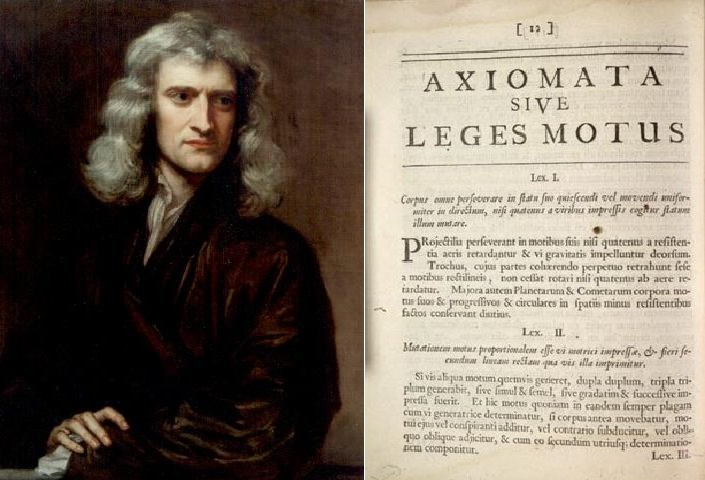# What are Newton’s Laws of Motion

Newton’s laws of motion are a set of three laws governing the motion of bodies, first published by Sir Isaac Newton (1643-1727) in 1687, in his famous publication,  Philosophiæ Naturalis Principia Mathematica. These laws form the cornerstone of dynamics in classical physics.

Newton’s laws of motion were built upon previous work by the famous Italian physicist Galileo Galilei (1564-1642) and the French philosopher René Descartes (1596-1650). In particular, Newton’s first law of motion is remarkably similar to Descartes’ Laws of Nature.

There are many different ways to state Newton’s Laws, but all of them cover the same principles.

## Newton’s First Law of Motion

A body continues to travel at a constant velocity as long as there is no resultant force acting on the body.

An alternative statement to describe Newton’s first law is: A body at rest stays at rest, or a body in motion continues to move at the same speed in a straight line, unless compelled by an external force.

## Newton’s Second Law of Motion

When a resultant force acts on a body, the body’s acceleration due to the resultant force is directly proportional to the force.

An alternative statement to describe Newton’s second law is: The resultant force on a body is equal to the body’s rate of change of momentum.

## Newton’s Third Law of Motion

If a body A exerts a force on body B, then the body B exerts a force of equal magnitude, in the opposite direction, on the body A.

An alternative statement to describe Newton’s third law is: Every action has a reaction, which has the same size and acts in the opposite direction.Newton’s laws of motion are not universally applicable. Technically speaking, Newton’s laws of motion are only valid for so-called “inertial reference frames” in physics. Issues also come up when bodies begin to travel closer to the speed of light, when masses of bodies begin to increase. Special relativity needs to be utilized to carry out calculations in these situations.  At very small scales, quantum mechanics needs to be used to understand the behaviour of particles. However, for everyday calculations, Newton’s laws of motion give answers to very good approximations. Using Newton’s laws of motion is also much easier compared to using more general, but complex theories.

References

Bain, J. (n.d.). Decartes & Newton. Retrieved 07 21, 2015, from Research|NYU Polytechnic School of Engineering: http://ls.poly.edu/~jbain/mms/handouts/mmsdescarteslaws.htm